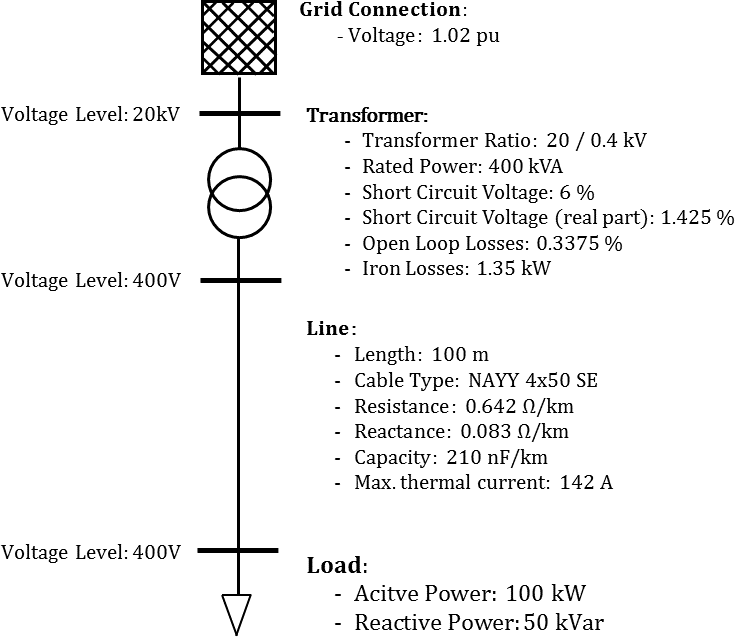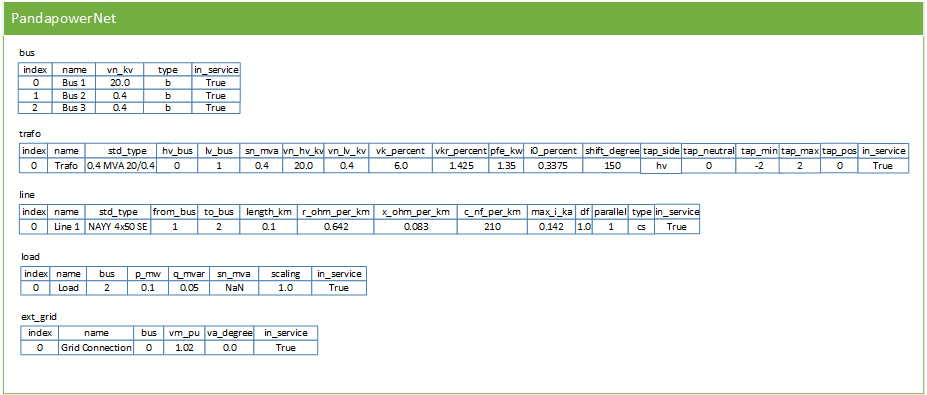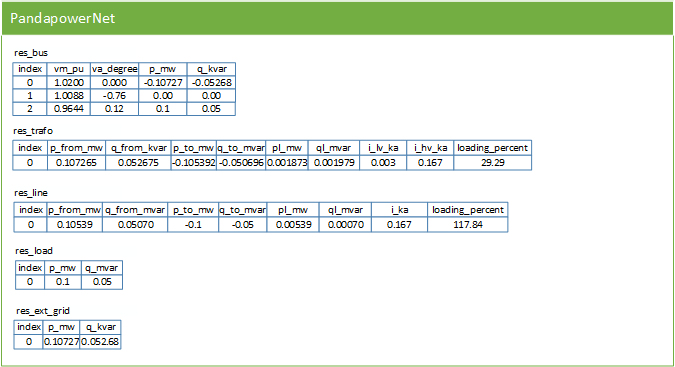# Getting Started

## Installing Python

pandapower is tested with Python 3.5, 3.6 and 3.7. We recommend the Anaconda Distribution, which provides a Python distribution that already includes a lot of modules for scientific computing that are needed. Simply download and install the newest version of Anaconda and you are all set to run pandapower.

Of course it is also possible to use pandapower with other distributions besides Anaconda. It is however important that the following packages are included:

• numpy
• scipy
• numba
• matplotlib

Since these packages depend on C-libraries, they cannot be easily installed through pip on Windows systems. If you use a distribution that does not include one of these packages, you either have to build these libraries yourself or switch to a different distribution.

## Installing pandapower

### Through pip

The easiest way to install pandapower is through pip:

1. Open a command prompt (e.g. start–>cmd on windows systems)

2. Install pandapower by running:

`````` pip install pandapower
``````

### Without pip

If you don’t have internet access on your system or don’t want to use pip for some other reason, pandapower can also be installed without using pip:

1. Download and unzip the current pandapower distribution from PyPi under “Download files”.
2. Open a command prompt (e.g. Start-->cmd on Windows) and navigate to the folder that contains the setup.py file with the command cd <folder> :

``````cd %path_to_pandapower%\pandapower-x.x.x\
``````
3. Install pandapower by running :

``````python setup.py install
``````

### Development Version

To install the latest development version of pandapower from github, simply follow these steps:

1. Download and install git.

2. Open a git shell and navigate to the directory where you want to keep your pandapower files.

3. Run the following git command:

`````` git clone https://github.com/e2nIEE/pandapower.git
``````
4. Navigate inside the repository and check out the develop branch:

`````` cd pandapower
git checkout develop
``````
5. Open a command prompt (cmd or anaconda command prompt) and navigate to the folder where the pandapower files are located. Run:

`````` pip install -e .
``````

This registers your local pandapower installation with pip.

## Test your installation

A first basic way to test your installation is to import all pandapower submodules to see if all dependencies are available:

``````import pandapower
import pandapower.networks
import pandapower.topology
import pandapower.plotting
import pandapower.converter
import pandapower.estimation
``````

If you want to be really sure that everything works fine, run the pandapower test suite:

1. Install pytest if it is not yet installed on your system:

``````pip install pytest
``````
2. Run the pandapower test suite:

`````` import pandapower.test
pandapower.test.run_all_tests()
``````

If everything is installed correctly, all tests should pass or xfail (expected to fail).

## A short introduction

A network in pandapower is represented in a pandapowerNet object, which is a collection of pandas Dataframes. Each dataframe in a pandapowerNet contains the information about one pandapower element, such as line, load transformer etc.

We consider the following simple 3-bus example network as a minimal example:### Creating a network

The above network can be created in pandapower as follows:

``````import pandapower as pp
#create empty net
net = pp.create_empty_network()

#create buses
b1 = pp.create_bus(net, vn_kv=20., name="Bus 1")
b2 = pp.create_bus(net, vn_kv=0.4, name="Bus 2")
b3 = pp.create_bus(net, vn_kv=0.4, name="Bus 3")

#create bus elements
pp.create_ext_grid(net, bus=b1, vm_pu=1.02, name="Grid Connection")
pp.create_load(net, bus=b3, p_mw=0.1, q_mvar=0.05, name="Load")

#create branch elements
tid = pp.create_transformer(net, hv_bus=b1, lv_bus=b2, std_type="0.4 MVA 20/0.4 kV", name="Trafo")
pp.create_line(net, from_bus=b2, to_bus=b3, length_km=0.1, name="Line",std_type="NAYY 4x50 SE")
``````

Note that you do not have to calculate any impedances or tap ratio for the equivalent circuit, this is handled internally by pandapower according to the pandapower transformer model. The standard type library allows comfortable creation of line and transformer elements.

The pandapower representation now looks like this:### Running a Power Flow

A powerflow can be carried out with the runpp function:

``````pp.runpp(net)
``````

When a power flow is run, pandapower combines the information of all element tables into one pypower case file and uses pypower to run the power flow. The results are then processed and written back into pandapower:For the 3-bus example network, the result tables look like this:All other pandapower elements and network analysis functionality (e.g. optimal power flow, state estimation or short-circuit calculation) is also fully integrated into the tabular pandapower datastructure.

This minimal example is also available as a jupyter notebook.

## Interactive Tutorials

There are jupyter notebook tutorials on different functionalities of pandapower:

Basic introduction:

Data analysis and modelling error diagnostic:

Optimal power flow:

State estimation:

Short-circuits:

Topology package:

Plotting pandapower networks (static with matplotlib):

Plotting pandapower networks (interactive with plotly)

Time series calculation with the pandapower control module

## YouTube Tutorials

You can also find some tutorials about the basics of pandapower on YouTube:

pandapower on YouTube